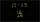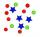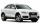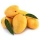# The kennel

20 dogs. Ratio dogs to cats is 3:2  how many cats?

c =  13.3333

### Step-by-step explanation:Did you find an error or inaccuracy? Feel free to write us. Thank you!Tips to related online calculators
Need help to calculate sum, simplify or multiply fractions? Try our fraction calculator.
Check out our ratio calculator.

## Related math problems and questions:

• The ratioThe ratio of girls to boys in Mrs. White's class is 3:2.  If there are 12 boys, how many girls are in the class room?
• Fractions and mixed numerals(a) Convert the following mixed numbers to improper fractions. i. 3 5/8 ii. 7 7/6 (b) Convert the following improper fraction to mixed number. i. 13/4 ii. 78/5 (c) Simplify these fractions to their lowest terms. i. 36/42 ii. 27/45 2. evaluate following ex
• ErikThree friends Peter, Erik and Milan shared 420 euros in a ratio of 1: 2: 3. How many euros did Erik get?
• Change the numbers in the ratioChange the numbers 29, 38, and 43 in a 3: 4 ratio.
• What is 9What is the value of x in the proportion 2 and one-fourth over x = 1 and one-half over 3 and three-fifths? 2 and two-fifths 5 and two-fifths 8 and 1 over 10 12 and 3 over 20
• Ratio - proportionReduce the number 16 in proportion 3:2 5:4 11:8
• Ratio three numbersThree numbers SUV are in the ratio 1:2:3. Their sum is 24. Find this numbers and write their add and sum.
• RatiosDivide: a) 250 CZK in the ratio 2:3 b) 1000 CZK in the ratio 4:7:9
• The product 2The product of two functions is 10. If one of them is 2 1/3, find the other one.
• Hours 3How many hours are in 3 2/3 days?
• Simplify expression with mixedWhen (7¹/3 + 2¾)÷(2 + 2¼ × 1¹/3) is simplified the result is?A basket is full of 50 different kinds of fruits that has a ratio of 2:3:5. If the fruits are apple, mango, and guava, how many guavas are there?The age of father and son is in the ratio 10: 3. The age of the father and daughter is in a ratio of 5: 2. How old are a father and a son if the daughter is 20?Which one of the following fraction after reducing in simplest form is not equal to 3/2? a) 15/20 b) 12/8 c) 27/18 d) 6/4Janko is 14 years old. The age ratio of Janka and Zuzka is 2: 3. What was the ratio seven years ago?The sum of 3 mixed numbers is 20 13/15. two of the numbers are 6 1/3 and 7 5/6. what is the third number?The machine was in operation for 9 tenths (in a fraction) of eight hours of working time. How long has he been running?